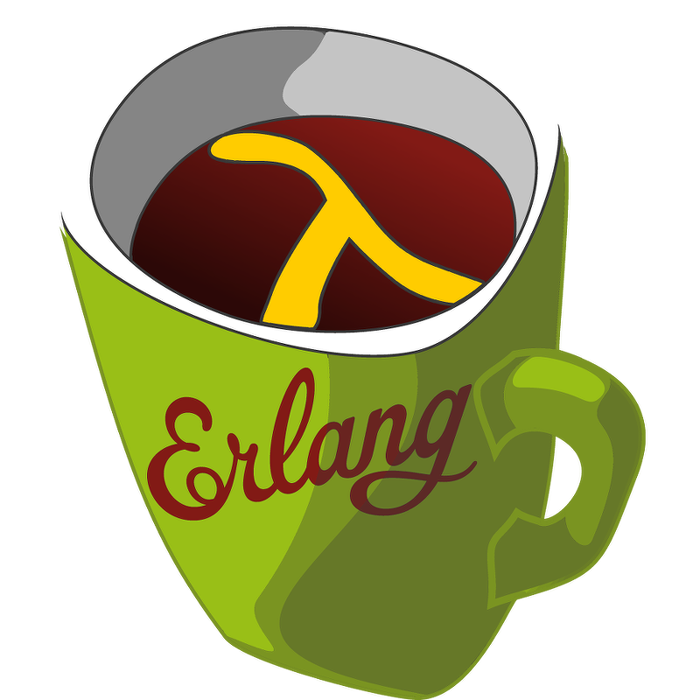This week's LFE Friday was translated with permission from the Erlang Thursday series by Steven Proctor. This week's translator: Robert Virding.

In last week's LFE Friday we continued to explore `ets:select/2` and seeing its use when combined with using `ets:fun2ms` to generate the `match_spec()`s.

This week we will take a look at the other versions of select that the `ets` module provides.

Yet again we will do our new playground ETS table setup, so if we crash our shell session we don't loose the table.

``````> (set fun (lambda () (receive (after 'infinity 'ok))))
#Fun<lfe_eval.23.88887576>
> (set some-process (spawn fun))
<0.36.0>
> (set test-table (ets:new 'test-table '(public)))
8207
> (ets:give_away test-table some-process ())
true``````

Next we will load our test ETS table with a bunch of test "products". For ease of example, we will just use a number for the product id, and a random price ending in `.99`.

``````> (list-comp ((<- product-id (lists:seq 1 10000)))
(ets:insert test-table `#(,product-id ,(+ (random:uniform 100) 0.99))))
(true true true true true true true true true true true true true
true true true true true true true true true true true true true
true true true true ...)``````

We will create a `match_spec()` to find items in their twenties (and we will go ahead and round 19.99 up to 20 just because).

``````> (set products-in-the-twenties (match-spec
([`#(,product ,price)]
(when (>= price 19.99) (< price 30))
`#(,product ,price))))
(#(#(\$1 \$2) (#(>= \$2 19.99) #(< \$2 30)) (#(#(\$1 \$2)))))``````

And if we use `ets:select/2` against our table with this match spec, we get all of the results back in one query as we saw previously.

``````> (ets:select test-table products-in-the-twenties)
(#(5671 24.99)
#(1322 23.99)
#(9221 24.99)
#(7109 23.99)
#(1792 20.99)
#(1659 29.99)
#(6565 19.99)
#(5151 24.99)
#(7655 22.99)
#(962 27.99)
#(9091 24.99)
#(7636 19.99)
#(1935 27.99)
#(4673 21.99)
#(3783 22.99)
#(1252 22.99)
#(2837 25.99)
#(5300 25.99)
#(2514 23.99)
#(8947 24.99)
#(7025 26.99)
#(289 25.99)
#(5954 23.99)
#(5302 19.99)
#(6670 22.99)
#(7398 22.99)
#(4022 27.99)
#(8047 20.99)
#(6569 ...)
#(...) ...)``````

But the `ets` module also gives us a way to limit the results if we would like, using select/3 and giving a limit of the number of results to return at a time.

So let's use `ets:select/3` and give a limit of `10` and see what happens.

``````> (ets:select test-table products-in-the-twenties 10)
#((#(5629 20.99)
#(278 20.99)
#(5016 26.99)
#(2642 19.99)
#(7347 28.99)
#(6429 28.99)
#(5415 23.99)
#(9022 19.99)
#(2422 23.99)
#(2806 21.99))
#(8207 960 10 #B() (#(9295 19.99)) 1))``````

We get a tuple back instead of a list of results. The first item in the tuple is a list of our first ten results we specified, the second is some bizarre looking tuple, which if we look at the documentation for `ets:select/3` represents something referred to as a continuation.

So we run our query again, and bind the results this time.

``````> (set `#(,results ,continuation) (ets:select test-table products-in-the-twenties 10))
#((#(5629 20.99)
#(278 20.99)
#(5016 26.99)
#(2642 19.99)
#(7347 28.99)
#(6429 28.99)
#(5415 23.99)
#(9022 19.99)
#(2422 23.99)
#(2806 21.99))
#(8207 960 10 #B() (#(9295 19.99)) 1))``````

So we have this continuation, but what is it and what does it mean for us to have it.

In short, it can be thought of as an immutable bookmark. It represents not only what page we are in for our query results, but also the book we are reading (our query).

This allows us to quickly pick up where we previously left off in our results set by passing the continuation to ets:select/1.

``````> (ets:select continuation)
#((#(7769 28.99)
#(5990 25.99)
#(885 23.99)
#(2105 27.99)
#(686 25.99)
#(8798 28.99)
#(9313 29.99)
#(969 26.99)
#(2965 22.99)
#(9295 19.99))
#(8207 577 10 #B() () 0))``````

And because it is our special immutable bookmark, every time we use that bookmark it takes us to the same starting point in the same book, and we only read the same number of maximum pages as originally set as our limit.

So no matter how many times we call `ets:select/1` with our same continuation, we will get the same results each time.

``````> (ets:select continuation)
#((#(7769 28.99)
#(5990 25.99)
#(885 23.99)
#(2105 27.99)
#(686 25.99)
#(8798 28.99)
#(9313 29.99)
#(969 26.99)
#(2965 22.99)
#(9295 19.99))
#(8207 577 10 #B() () 0))
> (ets:select continuation)
#((#(7769 28.99)
#(5990 25.99)
#(885 23.99)
#(2105 27.99)
#(686 25.99)
#(8798 28.99)
#(9313 29.99)
#(969 26.99)
#(2965 22.99)
#(9295 19.99))
#(8207 577 10 #B() () 0))
> (ets:select continuation)
#((#(7769 28.99)
#(5990 25.99)
#(885 23.99)
#(2105 27.99)
#(686 25.99)
#(8798 28.99)
#(9313 29.99)
#(969 26.99)
#(2965 22.99)
#(9295 19.99))
#(8207 577 10 #B() () 0))``````

And if we look at the resulting tuple, we see that we get a different tuple for our next continuation.

``````> (set `#(,2nd-results ,2nd-continuation) (ets:select continuation))
#((#(7769 28.99)
#(5990 25.99)
#(885 23.99)
#(2105 27.99)
#(686 25.99)
#(8798 28.99)
#(9313 29.99)
#(969 26.99)
#(2965 22.99)
#(9295 19.99))
#(8207 577 10 #B() () 0))``````

And we can pick up that new continuation, and use that in our next call to `ets:select/1` to get the next set of results, with another continuation.

``````> (ets:select 2nd-continuation)
#((#(3413 27.99)
#(2302 28.99)
#(1368 19.99)
#(3311 26.99)
#(415 24.99)
#(8068 23.99)
#(6664 29.99)
#(4920 24.99)
#(1979 20.99)
#(2091 21.99))
#(8207 897 10 #B() (#(5835 21.99) #(6629 24.99) #(9267 19.99)) 3))``````

And if we have a query for which we have exhausted our results set, we get an `\$end_of_table` atom.

``````> (ets:select test-table '(#(#(\$1 \$2) (#(< \$2 0)) (\$\$))) 10)
\$end_of_table``````

The ability to specify a limit and have a continuation is also available via on match with `ets:match/3` and `ets:match/1`, and match_object via `ets:match_object/3` and `ets:match_object/1`.

Next week, we will continue looking at the various `select` functions in `ets` as we look into their behavior with and ordered set, will look at `select` vs `select_reverse`, and play with and see how continuations work if we get some new entries inserted in the results when using a continuation.

- Proctor, Robert

23 January 2016

tutorials